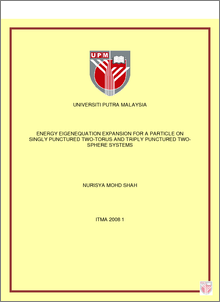# Energy Eigenequation Expansion for a Particle on Singly Punctured Two-Torus and Triply Punctured Two-Sphere Systems

## Citation

Mohd Shah, Nurisya (2008) Energy Eigenequation Expansion for a Particle on Singly Punctured Two-Torus and Triply Punctured Two-Sphere Systems. Masters thesis, Universiti Putra Malaysia.

## Abstract

Ideas from topology have played a major role in physics especially to describe and explain exotic quantum phenomena. There has been a considerable interest among physicists who are working on string theory and quantum gravity to use ideas and results from topology to explain their work. However, often one is limited to the choice of spaces with relatively simpler topologies e.g. sphere, torus etc because more complex spaces are difficult to be characterized or even distinguished. It is our particular interest to consider singularities (i.e. having one or several punctures on it) as a tool to generate a family of complex two-dimensional configuration surfaces. These surfaces may find applications in to mathematical models of quantum chaos, cosmology, particle physics, condensed matter, quantum gravity and string theory. Extensive mathematical studies have been carried out for punctured surfaces but their literature in physics are scarce. Most have tackled the case of quantum mechanical systems on punctured torus with respect to its scattering and chaotic behavior. Of particular interest in the present work are the quantum mechanical systems of singly punctured two-torus and triply punctured two-sphere. They both have two generators and three possible non-contractible loops. Both surfaces can be generated from the same parent generators of the modular group Γ, which is a discrete subgroup of linear fractional transformations of the upper half complex plane H (the universal cover of the punctured surfaces). In this dissertation, we construct both surfaces of singly punctured two-torus and triply punctured two-sphere stepwise using these generators. The main aim however is to construct the energy eigenequation for particle on surfaces of singly punctured two-torus and triply punctured two-sphere. For that purpose, we first identify the configuration space explicitly by considering the tessellation of the upper half-plane and the required surfaces are determined. Next, by using the Fourier expansions, finite Fourier transform of the energy eigenequation is performed to give rise to a sought standard relation for generating the eigenfunction. It is known that the eigenfunction on a punctured system exhibit both discrete and continuous energy spectra. The discrete energy spectrum will correspond to the computation of a countable number of Maass cusp forms while for the continuous spectrum, it is spanned by the Eisenstein series. In this work, we present the expressions for the Maass cusp forms of the singly punctured two-torus and triply punctured two-sphere and the expression of the Eisenstein series for the singly punctured two-torus. At the end of this thesis a unified treatment of the Maass cusp forms and the Eisensteins series for the singly punctured two-torus and the triply punctured two-sphere are presented. The importance of each technique used on the formation of the energy eigenequation are explained in a more physical approach.Preview
PDF
ITMA_2008_1.pdfView Item